# Tributaries

We can fill the pool with two different tributaries. The first inflow would fill the pool in 18 hours, both in 6 hours. How many hours would the pool fill with a second inflow?

t2 =  9 h

### Step-by-step explanation:Did you find an error or inaccuracy? Feel free to write us. Thank you!Tips to related online calculators
Need help to calculate sum, simplify or multiply fractions? Try our fraction calculator.
Do you have a linear equation or system of equations and looking for its solution? Or do you have a quadratic equation?
Tip: Our volume units converter will help you with the conversion of volume units.
Do you want to convert time units like minutes to seconds?

## Related math problems and questions:

• Tributaries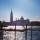The first tributary fill pool with water in 15 hours. The second tributary fill pool in 10 hours. For how many hours the pool is filled with both tributaries?
• Two tributaries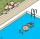Two tributaries of the pool fill it in 10 hours. One of the tributaries would fill 15 hours. How long would fill the first tributary?
• Pool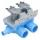Pool is filled with two water supply. The first supply fill pool for nine hours, the second for six hours. How many hours will take fill the pool when the water flows in through the first supply 3 hours and then we will open a second supply?
• Three tributaries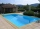It is possible to fill the pool with three tributaries. The first would take 12 hours, the second 15 hours, and the third 20 hours. The day before the summer season began, the manager opened all three tributaries simultaneously. How long did it take to fi
• Pool 2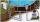The first supply by the pool fill for five hours and the second fill for six hours, drain should be drained for 15 hours. For how many hours the pool is full, when we open both inlet now and outlet open two hours later?
• Three pumpsWe are filling the pool. The first pump would be filled in 12 hours, the second pump in 15 hours. If all three pumps were running at the same time, it would fill the pool for 4 hours. How long would the pool fill only with the third pump?
• Sprayers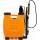The vineyard can be sprayed first sprayer in 5 hours and with the second in 8 hours. How long would it take if we use sprayers both at once?
• Work togetherTwo bricklayers plastering a wall. The first would plaster it in 8 hours, the second in 12 hours. How many hours will they be done with the work if they work together?
• Two workersThe first worker would himself completed the work in 8 hours, the other in 6 hours. After two hours of joint work, the worker left the first worker to a doctor, and others completed the work himself. How many hours worked the other worker himself?
• Two looms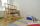On two different looms with different performance, the required quantity of textile in 6 hours when both work together. On the first machine, this quantity of the textile can be made in 10 hours. How long should it take to make the same quantity of textil
• The factoryThe factory z can complete an order in 12 days, factory p in 18 days. How many days does an order completed when the first two days work only the factory z and then both factories?
• PoolIf water flows into the pool by two inlets, fill the whole for 19 hours. The first inlet filled pool 5 hour longer than the second. How long pool take to fill with two inlets separately?
• Pumps 3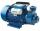Two pumps of the same power fill the garden pool for 10 hours. How many of these pumps would have to use if we want to shorten the filling of the pool to four hours?
• The dam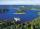The water reservoir is filled with first tributary for 25 hours second tributary for 35 hours. Some time were both inlets open, then a second tributary closed and the tank was filled in four hours. What time water flowed from both tributaries? (Expressed
• How many 15How many six-eights are there in 18? Which of the following would you choose to solve this question? 18 x 6/8 or 18 : 6/8
• Water pool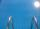Pool with volume 990hl completely filled, if water flows by one tap 8 hours and by second tap 6 hours. First tap give 10hl more than second per hour. How many hl flows in each of them in an hour?
• Parquet floor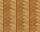Three workers laid the parquet floor for 2.301 hour. The first one would do this work alone 7 hours, the second in 6 hours. For how many hours would fulfill this work the third worker if he worked alone?